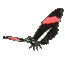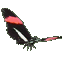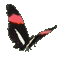Teaching Trigonometry Tangents - Fun Math Help - Game Tips:

- The tangent of angle A can be written as tan A and equals the slope from point A(0,0) to point D(2,4).
This tangent ratio = slope = (rise)/(run) = (change in y)/(change in x) = (4-0)/(2-0) = (4)/(2) = 2/1.
Notice the rise is perpendicular to the run.

- Generally, when angle A is in standard position as shown at the graph's origin (0,0)
then tan A equals the ratio = (rise)/(run) = slope.
Also in any right triangle, tan A equals (opposite)/(adjacent) = (y)/(x).

- Your Game Score is reduced by the number of butterfly hits.

- To slow the game speed repeat tap/click on the word Slider.
- To increase the game speed repeat tap/click on the word Math.
- Speed can also be adjusted with a keyboard's - and + keys.

- Refresh/Reload the web page to restart the game.